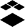# New Quizzes: MathQuill for special numerical entries

When choosing the numerical entry question in new quiz, the mathquill does not populate thus not allowing students to enter exponents, fractions, radicals etc as numerical answers.  Please fix this.Instructure Alumni
Status changed to: OpenCommunity Member

Students should have the ability to use the equation editor in a numerical answer, or at the very least, be able to use the backslash (/) to give a fractional answer.Community Member

The equation editor is not available in the answer, that is the problem.  Fractions are easy as you mentioned, however radicals and other irrational numbers cannot be entered.Community Participant

Please add this ability. I know this is a big ask but if canvas can grade general math expressions I will not need to ask my students to pay for MyLab any more.Community Member

Mathquill toolbar needs a fraction and a division bracketCommunity Member

also mixed numbers, make it similar to the classic tool barCommunity Novice

It would be appreciated if you could use fractions in a numerical response question as a possible answer